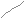Archive for the ·

Python

· Category...

Sparklines

Looking for a Python sparkline library, I found Perry Geo’s excellent code. “In the minimalist spirit of sparklines, the interface was kept simple”:

```import spark a = [32.5,35.2,39.9,40.8,43.9,48.2,50.5,51.9,53.1,55.9,60.7,64.4] spark.sparkline_smooth(a).show()```

That’s it, and here’s the result.Just download his single Python module, start up interactive Python, and off you go.

This of course sent me on a tangent, off to Edward Tufte’s work and creation of sparklines. It seems I have a book or two to buy.

A note about multiple inheritance in Python

Consider the following three classes, where C inherits from A and B, and the method m() is present in both A and B.

``````
class A(object):
def m(self):
print "a.m"

class B(object):
def m(self):
print "b.m"

class C(A, B):
def test(self):
pass
``````

Now, if you create an instance of class C and invoke method m, what will be the result?

``````
>>> c = C()
>>> c.m()
a.m
``````

The Python docs tutorial on the subject says “the resolution rule used for class attribute references (…) is depth-first, left-to-right.”, which seems like an easy rule. However, it also warns about some maintenance nightmares, e.g “a class derived from two classes that happen to have a common base class”.

Class attributes in Python

After almost two hours of debugging, I finally made an interesting (at least to me) discovery in Python: Attributes of type dictonary and list (and presumably all other object types) at the class level are “static” (as used in Java classes), while primitive types are not. Does that make sense? I don’t know.

Consider the following dummy class:
``` ```

``````class MyClass:
e = {"a":None}
f = 
i = 50
def __init__(self):
self.d = {"a":None}
def set(self, v):
self.d["a"] = v
self.e["a"] = v
self.f = v
``````
``` ```

We have four attributes here:
e – a class level dictionary
f – a class level list
i – an int
d – another dictionary referenced by self.d inside the methods.

Now consider the following result from these interactive lines:
``` ```

``````>>> x = MyClass()
>>> x.set(5)
>>> x.d
{'a': 5}
>>> x.e
{'a': 5}
>>> x.f

>>> y = MyClass()
>>> y.set(10)
>>> x.d
{'a': 5}
>>> x.e
{'a': 10}
>>> x.f

>>>
>>> y.i = 100
>>> x.i
50
>>> y.i
100
>>>
``````
``` ```

As you can see, the first call to set method, set the value 5 in x.e, x.f and x.d. However, the second call, y.set(10), will also set the value of x.e and x.f (in red). Finally, it is shown that the int i does not conform to this behaviour.

Strange, I say.